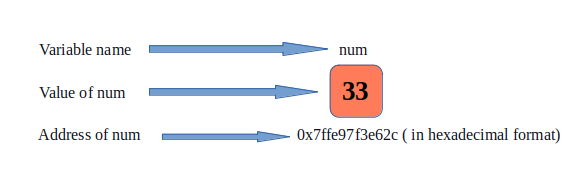# How to Print Address in C

In this article, we will first look at how can we assign an address to a variable and then print the address using C language.

First of all, we will take a look at how addresses are assigned to any variable.

Whenever we declare a variable of any data type (int, float, double, char, etc.), some memory is allocated to the variable by the operating system which can be any random number but cannot be negative. This random number is called the address of that variable and every variable has a unique address.

To understand this concept in a more simple manner, let us take one real-life example. Suppose Rahul is a student of some university and lives in Hostel B, Room Number 32. Now, if someone asks me – Who lives in 32B? My prompt would be Rahul. Here, 32B is the address of a value named Rahul. Similar to this nature, addresses are assigned to variables in computer programs too.

There exist two methods to print the address of a variable in C :

• Using address of (&), also called as ampersand operator.

• Using pointers in C.

To print any value in a program, C language uses the ‘printf’ function. The format to print output in C is given as – printf(“<format specifier>”, <variable to be printed>).

The address of a variable is an integer numeric quantity and the format specifier used to print such a quantity is “%p” (for printing address in hexadecimal form) and “%lld” (for printing address in decimal form). To print the address of a variable, we use ‘&’ along with the variable name.

Let us understand this with a sample program:

Output:

In this program, our variable name is ‘num’. To print its address, the format specifier used here is ‘%p’ and ‘%lld’ and ‘&’ operator is used along with the name of the variable. The second print statement is used to insert a line break between the two outputs. Note that the output that gives the address of this variable is a randomly generated quantity. It can also take any other value than the one mentioned above.## Method 2: Printing Address Using Pointer

The address of a variable can also be printed using pointers in C. A pointer is an integer component that stores the address of another variable. To use a pointer, it must also be declared just like any other variable. A pointer in C is declared using the ‘*’ operator, also called the asterisk operator.

Pointer declaration – <datatype> *<variable_name>. Here, variable_name is used to store the address of the other variable.

Let us understand this with a sample program:

Output:

In this program, a pointer ‘p’ is initialized which stores the address of the variable ‘num’. As we have seen earlier, the ampersand operator is used to access the address of a variable, we use the ‘address of’ operator and store the variable’s address in ‘p’. Now, to print the address of the variable, we use the printf function along with the format specifier and the variable to be printed ( i.e., ‘p’ ).In this way, we can make use of any of the two methods to print the address of a variable.